# Engaging Math Lessons.

Please browse our selection of mathematics lessons sorted by subject and current common core standards for improving math achievement for every student.

Below are a set of lesson plans and worksheets for Grade 8 Math that correspond to Common Core concepts and help students solve real-world problems. Lesson previews provide a quick glimpse at the provided materials.

• ### Concept:

Expressions & Equations
• Common Core Standard: CCSS.Math.Content.8.EE.A.1
• Description: Know and apply the properties of integer exponents to generate equivalent numerical expressions.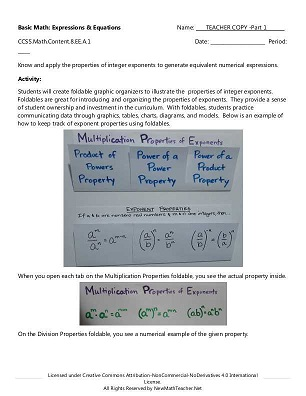• ### Concept:

Expressions & Equations
• Common Core Standard:CCSS.Math.Content.8.EE.A.2
• Description: Use square root and cube root symbols to represent solutions to equations of the form x2 = p and x3 = p, where p is a positive rational number. Evaluate square roots of small perfect squares and cube roots of small perfect cubes. Know that √2 is irrational.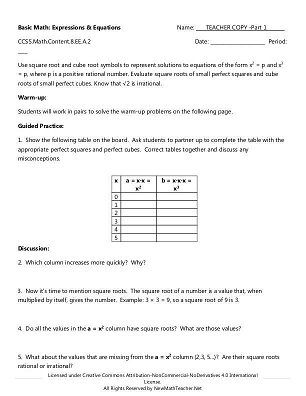• ### Concept:

Expressions & Equations
• Common Core Standard:CCSS.Math.Content.8.EE.A.3
• Description: Use numbers expressed in the form of a single digit times an integer power of 10 to estimate very large or very small quantities, and to express how many times as much one is than the other.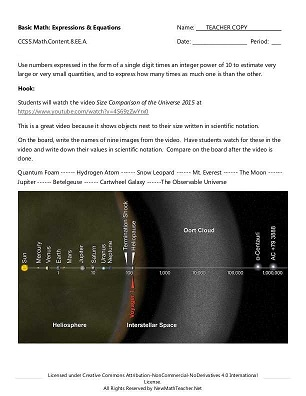• ### Concept:

Expressions & Equations
• Common Core Standard:CCSS.Math.Content.8.EE.A.4
• Description: Perform operations with numbers expressed in scientific notation, including problems where both decimal and scientific notation are used. Use scientific notation and choose units of appropriate size for measurements of very large or very small quantities. Interpret scientific notation that has been generated by technology.• ### Concept:

Expressions & Equations
• Common Core Standard:CCSS.Math.Content.8.EE.B.5
• Description: Graph proportional relationships, interpreting the unit rate as the slope of the graph. Compare two different proportional relationships represented in different ways.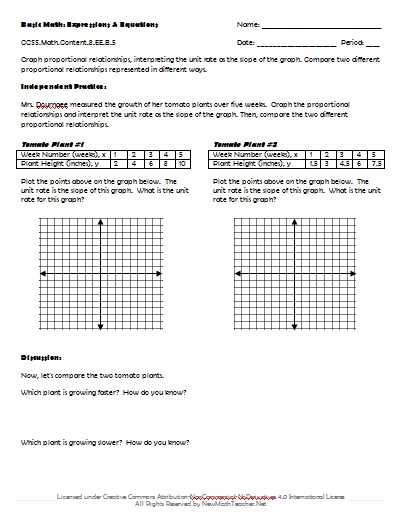• ### Concept:

Expressions & Equations
• Common Core Standard:CCSS.Math.Content.8.EE.B.6
• Description: Use similar triangles to explain why the slope m is the same between any two distinct points on a non-vertical line in the coordinate plane; derive the equation y = mx for a line through the origin and the equation y = mx + b for a line intercepting the vertical axis at b.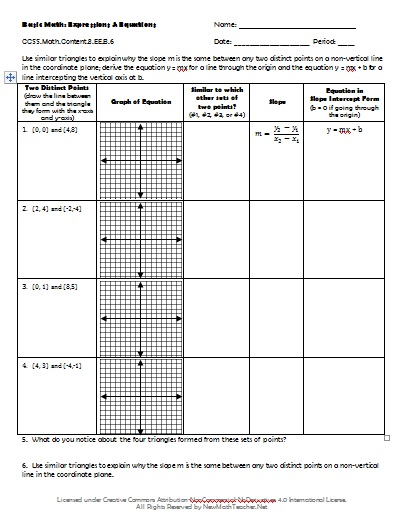• ### Concept:

Expressions & Equations
• Common Core Standard:CCSS.Math.Content.8.EE.C.7.A
• Description: Give examples of linear equations in one variable with one solution, infinitely many solutions, or no solutions. Show which of these possibilities is the case by successively transforming the given equation into simpler forms, until an equivalent equation of the form x = a, a = a, or a = b results (where a and b are different numbers).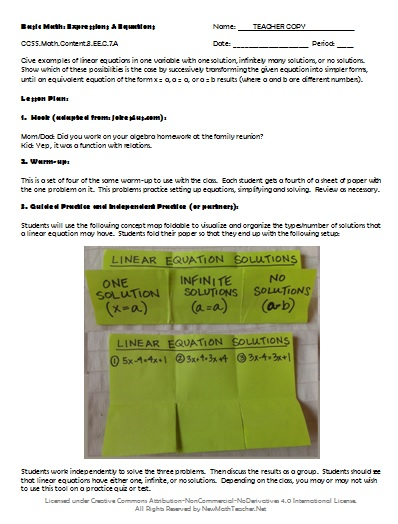• ### Concept:

Expressions & Equations
• Common Core Standard:CCSS.Math.Content.8.EE.C.7.B
• Description: Solve linear equations with rational number coefficients, including equations whose solutions require expanding expressions using the distributive property and collecting like terms.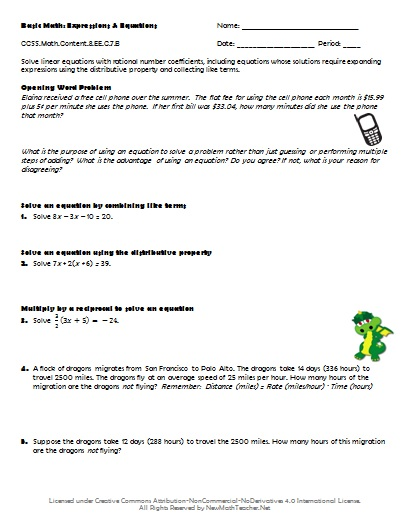• ### Concept:

Expressions & Equations
• Common Core Standard:CCSS.Math.Content.8.EE.C.8.A
• Description: Understand that solutions to a system of two linear equations in two variables correspond to points of intersection of their graphs, because points of intersection satisfy both equations simultaneously.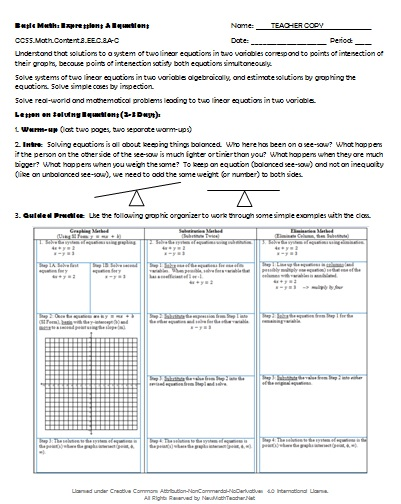• ### Concept:

Expressions & Equations
• Common Core Standard:CCSS.Math.Content.8.EE.C.8.B
• Description: Solve systems of two linear equations in two variables algebraically, and estimate solutions by graphing the equations. Solve simple cases by inspection.• ### Concept:

Expressions & Equations
• Common Core Standard:CCSS.Math.Content.8.EE.C.8.C
• Description: Solve real-world and mathematical problems leading to two linear equations in two variables.